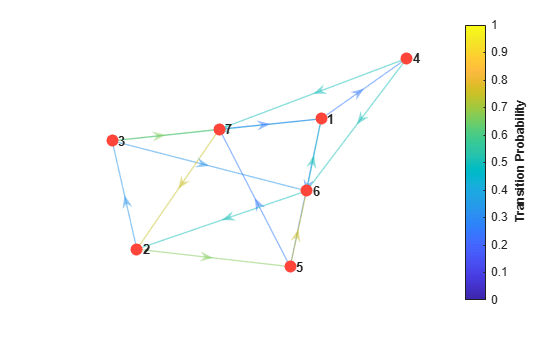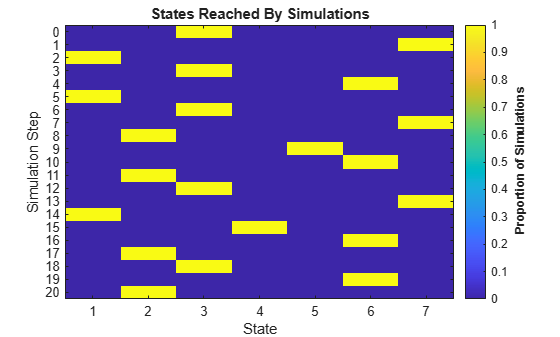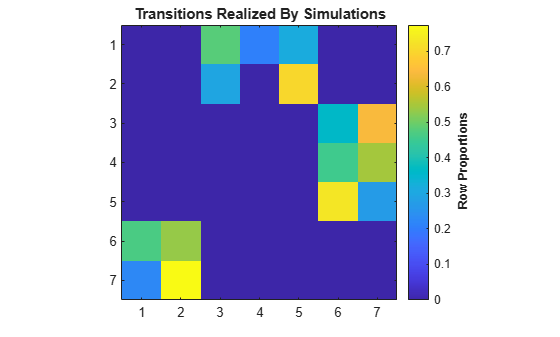# simplot

Plot Markov chain simulations

## Syntax

``simplot(mc,X)``
``simplot(mc,X,Name,Value)``
``simplot(ax,___)``
``h = simplot(___)``

## Description

example

``simplot(mc,X)` creates a heatmap from the data `X` on random walks through sequences of states in the discrete-time Markov chain `mc`.`

example

````simplot(mc,X,Name,Value)` uses additional options specified by one or more name-value arguments. For example, specify the type of plot or frame rate for animated plots.```
``` `simplot(ax,___)` plots on the axes specified by `ax` instead of the current axes (`gca`) using any of the input argument combinations in the previous syntaxes. The option `ax` can precede any of the input argument combinations in the previous syntaxes.```
````h = simplot(___)` returns the plot handle. Use `h` to modify properties of the plot after you create it.```

## Examples

collapse all

Consider this theoretical, right-stochastic transition matrix of a stochastic process.

`$P=\left[\begin{array}{ccccccc}0& 0& 1/2& 1/4& 1/4& 0& 0\\ 0& 0& 1/3& 0& 2/3& 0& 0\\ 0& 0& 0& 0& 0& 1/3& 2/3\\ 0& 0& 0& 0& 0& 1/2& 1/2\\ 0& 0& 0& 0& 0& 3/4& 1/4\\ 1/2& 1/2& 0& 0& 0& 0& 0\\ 1/4& 3/4& 0& 0& 0& 0& 0\end{array}\right].$`

Create the Markov chain that is characterized by the transition matrix P.

```P = [ 0 0 1/2 1/4 1/4 0 0 ; 0 0 1/3 0 2/3 0 0 ; 0 0 0 0 0 1/3 2/3; 0 0 0 0 0 1/2 1/2; 0 0 0 0 0 3/4 1/4; 1/2 1/2 0 0 0 0 0 ; 1/4 3/4 0 0 0 0 0 ]; mc = dtmc(P);```

Plot a directed graph of the Markov chain. Indicate the probability of transition by using edge colors.

```figure; graphplot(mc,'ColorEdges',true);```Simulate a 20-step random walk that starts from a random state.

```rng(1); % For reproducibility numSteps = 20; X = simulate(mc,numSteps)```
```X = 21×1 3 7 1 3 6 1 3 7 2 5 ⋮ ```

`X` is a 21-by-1 matrix. Rows correspond to steps in the random walk. Because `X(1)` is `3`, the random walk begins at state 3.

Visualize the random walk.

```figure; simplot(mc,X);```Consider this theoretical, right-stochastic transition matrix of a stochastic process.

`$P=\left[\begin{array}{ccccccc}0& 0& 1/2& 1/4& 1/4& 0& 0\\ 0& 0& 1/3& 0& 2/3& 0& 0\\ 0& 0& 0& 0& 0& 1/3& 2/3\\ 0& 0& 0& 0& 0& 1/2& 1/2\\ 0& 0& 0& 0& 0& 3/4& 1/4\\ 1/2& 1/2& 0& 0& 0& 0& 0\\ 1/4& 3/4& 0& 0& 0& 0& 0\end{array}\right].$`

Create the Markov chain that is characterized by the transition matrix P.

```P = [ 0 0 1/2 1/4 1/4 0 0 ; 0 0 1/3 0 2/3 0 0 ; 0 0 0 0 0 1/3 2/3; 0 0 0 0 0 1/2 1/2; 0 0 0 0 0 3/4 1/4; 1/2 1/2 0 0 0 0 0 ; 1/4 3/4 0 0 0 0 0 ]; mc = dtmc(P);```

Generate 100 20-step random walks starting from state 1.

```rng(1); % For reproducibility numSteps = 20; X0 = zeros(mc.NumStates,1); X0(1) = 100; % 100 random walks starting from state 1 only X = simulate(mc,numSteps,'X0',X0);```

`X` is a 21-by-100 matrix.

Visualize the proportion of times that states transition to other states for all random walks by using a heatmap.

```figure; simplot(mc,X,'Type','transitions');```Rows and columns of the heatmap correspond to state numbers.

Compare the realized transition matrix to the theoretical transition matrix by using a heatmap.

```figure imagesc(mc.P) axis square colorbar```The realized and theoretical transition matrices are similar.

## Input Arguments

collapse all

Discrete-time Markov chain with `NumStates` states and transition matrix `P`, specified as a `dtmc` object. `P` must be fully specified (no `NaN` entries).

Simulated data, specified as a `(1 + numSteps)`-by-`numSims` numeric matrix of positive integers returned by `simulate`. The first row contains the initial states. Columns represent random walks from the corresponding initial state.

Data Types: `double`

Axes on which to plot, specified as an `Axes` object.

By default, `simplot` plots to the current axes (`gca`).

### Name-Value Arguments

Specify optional pairs of arguments as `Name1=Value1,...,NameN=ValueN`, where `Name` is the argument name and `Value` is the corresponding value. Name-value arguments must appear after other arguments, but the order of the pairs does not matter.

Before R2021a, use commas to separate each name and value, and enclose `Name` in quotes.

Example: `'Type','Graph','FrameRate',3` creates an animated plot of the simulations using a frame rate of 3 seconds.

Plot type, specified as the comma-separated pair consisting of `'Type'` and a value in this table.

ValueDescription
`'states'`

States reached by simulations in `X`. The plot is a `(1 + numSteps)`-by-`NumStates` heatmap. Row i displays the proportion of walks in each state at step i.

`'transitions'`

Transitions realized by simulations in `X`. The plot is a `NumStates`-by-`NumStates` heatmap. Element (i,j) displays the proportion of transitions from state i to state j in all simulations. The plot is an empirical estimate of the transition matrix `mc.P`.

`'graph'`

Animated graph of state counts (node size) and state flows (edge width) at each step. The `'FrameRate'` name-value pair argument controls the animation progress.

Example: `'Type','graph'`

Data Types: `string` | `char`

Length of discrete time steps, in seconds, for animated plots, specified as the comma-separated pair consisting of `'FrameRate'` and a positive scalar.

The default is a pause at each time step. The animation proceeds when you press the space bar.

Example: `'FrameRate',3`

Data Types: `double`

## Output Arguments

collapse all

Handle to the simulation plot, returned as a graphics object. `h` contains a unique plot identifier, which you can use to query or modify properties of the plot.

## Tips

To compare a plot of realized transitions (`'Type','transitions'`) with the transition matrix, use:

```figure imagesc(mc.P) axis square```

## Version History

Introduced in R2017b